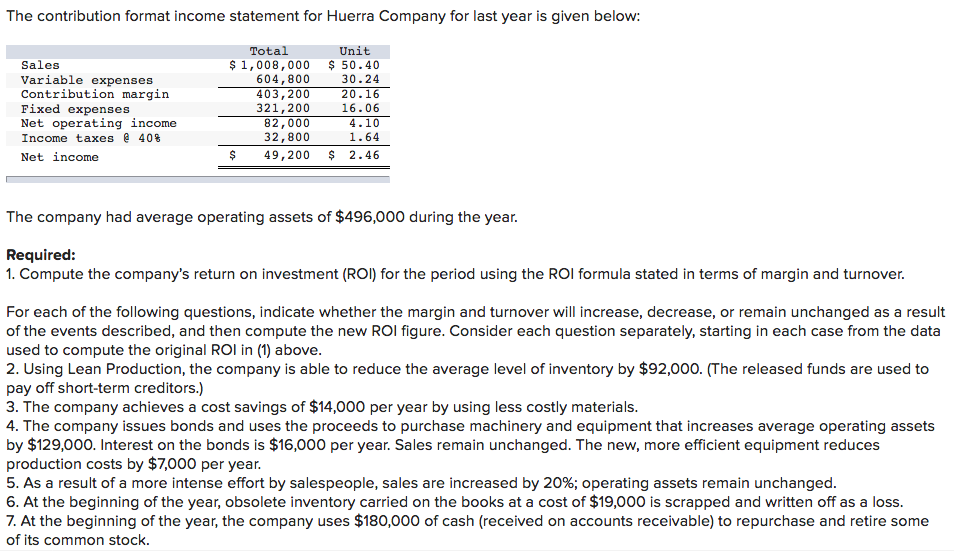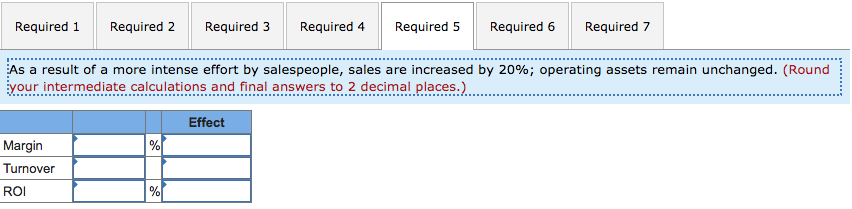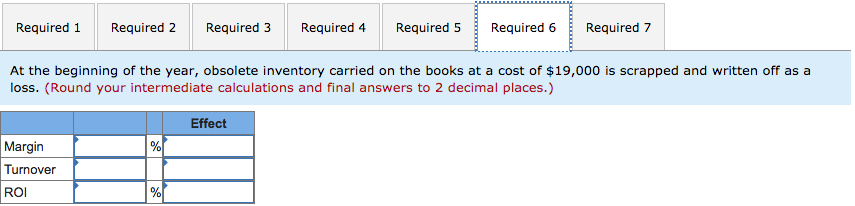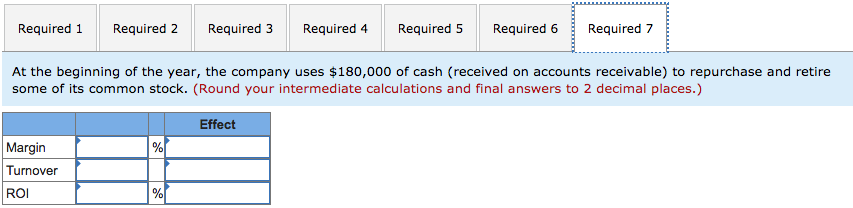# The contribution format income statement for Huerra Company for last year is given below: Total U...The contribution format income statement for Huerra Company for last year is given below: Total Unit Sales Variable expenses Contribution marain Fixed expenses Net operating income Income taxes @ 40% Net income \$ 1,008,000 50.40 30.24 20.16 16.06 4.10 1.64 \$ 49,200 2.46 604,800 403,200 321,200 82,000 32,800 The company had average operating assets of \$496,000 during the year. Required: 1. Compute the company's return on investment (ROI) for the period using the ROI formula stated in terms of margin and turnover For each of the following questions, indicate whether the margin and turnover will increase, decrease, or remain unchanged as a result of the events described, and then compute the new ROI figure. Consider each question separately, starting in each case from the data used to compute the original ROl in (1) above 2. Using Lean Production, the company is able to reduce the average level of inventory by \$92,000. (The released funds are used to pay off short-term creditors.) 3. The company achieves a cost savings of \$14,000 per year by using less costly materials 4. The company issues bonds and uses the proceeds to purchase machinery and equipment that increases average operating assets by \$129,000. Interest on the bonds is \$16,000 per year. Sales remain unchanged. The new, more efficient equipment reduces production costs by \$7,000 per year As a result of a more intense effort by salespeople, sales are increased by 20%; operating assets remain unchanged 6. At the beginning of the year, obsolete inventory carried on the books at a cost of \$19,000 is scrapped and written off as a loss 7. At the beginning of the year, the company uses \$180,000 of cash (received on accounts receivable) to repurchase and retire some of its common stock.
Required 1 Required 2 Required 3 Required 4Required 5 Required 6Required 7 As a result of a more intense effort by salespeople, sales are increased by 20%; operating assets remain unchanged. (Round your intermediate calculations and final answers to 2 decimal places) Effect Margin Turnover ROI
Required 1 Required 2 Required 3 Required 4 Required 5Required 6Required 7 At the beginning of the year, obsolete inventory carried on the books at a cost of \$19,000 is scrapped and written off as a loss. (Round your intermediate calculations and final answers to 2 decimal places.) Effect Margin Turnover ROI
Required 1 Required 2 Required 3 Required 4 Required 5 Required 6 Required 7 At the beginning of the year, the company uses \$180,000 of cash (received on accounts receivable) to repurchase and retire some of its common stock. (Round your intermediate calculations and final answers to 2 decimal places.) Effect Margin Turnover ROI

Rate of return, ROI = Profit margin x Asset turn over

= (Net operating income/Sales) x (Sales/Average operating assets)

1.

Profit margin = \$ 82,000/\$ 1,008,000 = 0.0813492063492064 or 8.13 %

Asset turn over = \$ 1,008,000/\$ 496,000 = 2.03225806451613 or 2.03

ROI = 8.13 % x 2.03 = 16.5039 % or 16.50 %

2.

Profit margin = \$ 82,000/\$ 1,008,000 = 0.0813492063492064 or 8.13 %    Unchanged

Asset turn over = \$ 1,008,000/ (\$ 496,000 - \$ 92,000) = \$ 1,008,000/\$ 404,000

= 2.4950495049505 or 2.50    Increased

ROI = 8.13 % x 2.50 = 20.325 % or 20.33 %   Increased

3.

Profit margin = (\$ 82,000+ \$ 14,000)/\$ 1,008,000 = \$ 96,000/\$ 1,008,000

=0.0952380952380952 or 9.52 %    Increased

Asset turn over = \$ 1,008,000/\$ 496,000 = 2.03225806451613 or 2.03   Unchanged

ROI = 9.52 % x 2.03 = 19.3256 % or 19.33 %   Increased

4.

Profit margin = (\$ 82,000 + \$ 7,000)/\$ 1,008,000 = \$ 89,000/\$ 1,008,000

= 0.0882936507936508 or 8.83 %   Increased

Asset turn over = \$ 1,008,000/ (\$ 496,000 + \$ 129,000) = \$ 1,008,000/\$ 625,000

= 1.6128 or 1.61   Decreased

ROI = 8.83 % x 1.61 = 14.2163 % or 14.22 %       Decreased

* Interest expense is finance activity and is not considered to compute net operating income.

5.

 Sales (\$1,008,000 x 1.2) \$ 1,209,600 Variable cost (\$ 604,800 x 1.2) \$ 725,760 Contribution margin \$ 483,840 Fixed expenses \$ 321,200 Net operating income \$ 162,640

Profit margin = \$ 162,640 / \$ 1,209,600 = 0.134457671957672 or 13.45 %   Increased

Asset turn over = \$ 1,209,600/\$ 496,000 = 2.43870967741935 or 2.44 Increased

ROI = 13.45 % x 2.44 = 32.818 % or 32.82 %   Increased

*** Answered for 4 +1 sub-parts against minimum 4 sub-parts of Chegg’s policy.

##### Add Answer of: The contribution format income statement for Huerra Company for last year is given below: Total U...
More Homework Help Questions Additional questions in this topic.